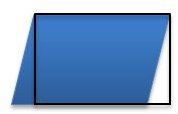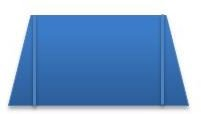# Find the Area by Decomposing Polygons: A 6th Grade Math Lesson

Page content

Find area of polygons by composing or decomposing polygons into triangles, rectangles, or other shapes.

Lesson Objective: The lesson is aligned to the Common Core State Standards for Mathematics – 6.G.1 Geometry – Find area of right triangles, other triangles, special quadrilaterals, and polygons by composing into rectangles or decomposing into triangles and other shapes; apply these techniques in the context of solving real-world and mathematical problems.

Materials Required: Calculator

## Lesson Procedure

Rectangles can be decomposed into two congruent right triangles as shown on the right.

The area of a right triangle is ½ the area of the rectangle. The area of a rectangle can be found by multiplying the base times the height; A = b x h. The area of a triangle is ½ x b x h.Look at the rectangle on the right. The measurement of the base is 6 units. The measurement of the height is 3 units.

1. What is the area of the rectangle?
2. What is the area of the triangle labeled “a”?
3. Describe how you found the area of the triangle.Finding the Area of an Isosceles Trapezoid

Isosceles trapezoids can be decomposed into two congruent right triangles and a rectangle as shown on the right.

The area of an isosceles trapezoid is the sum of the areas of the two right triangles and the area of the rectangle.Look at the isosceles trapezoid on the right. The measurements of the bases are 6 units and 10 units. The measurement of the height is 4 units.

1. Decompose the isosceles trapezoid into two congruent right triangles and a rectangle.
2. What is the area of the rectangle? Show how you found your answer.
3. What is the base of the right triangles? Show how you found your answer.
4. What is the area of the right triangles? Show how you found your answer.
5. What is the area of the isosceles trapezoid? Show how you found your answer.

1.18 square units

2. 9 square units

3. I found the area of the rectangle by multiplying the the measurement of the base times the measurment of the height. Then I took half of the product.

4. Base is 6 units and the height is 4 units. 6 x 4 = 24 square units.

5. The base of one right triangle is ½ of (10 – 6), 2 units.

6. The base of the right triangle is 2 units and the height is 4 units. The area of the right triangle is ½ x 2 x 4 = 4 square units.

7. Area of the two right triangles is 4 square units + 4 square units = 8 square units. The area of the isosceles trapezoid is the sum of the areas of the right triangles and the area of the rectangle is 8 square units + 24 square units = 32 square units.

## Individual or Group Work:Look at the figures below. Compose of decompose the figures into triangles and rectangles to find the areas of the figures.

1. The measurement of the base of the parallelogram is 8 units. The measurement of the height is 4 units. What is the area of the parallelogram? Show how you found your answer.2. The measurments of the bases of the isosceles trapezoid is 9 units and 15 units. The height of the trapezoid is 8 units. What is the area of the isosceles trapezoid? Show how you found your answer.3. The measurment of the base of the rectangle is 15 units. The measurement of the height is 6 units. What is the area of the right triangle labeled “a”? Show how you found your answer.1. To find the area of the parallelogram, I decomposed the parallelogram into two triangles and a rectangle. I moved the triangle on the left to the right side of the parallelogram to form one rectangle. The measure of the base is 8 units and the measure of the height is 4 units. The area is base x height, 4 x 8 = 32 square units.2. To find the area of the isosceles trapezoid, I decomposed the trapezoid into two congruent triangles and a rectangle. I found the base of the traingles by ½ (15 – 9) = 3. The area of one right triangle is ½ x base x height, ½ x 3 x 8 = 12 square units. The area of the rectangle is base x height, 9 x 8 = 72 square units. The area of the isosceles trapezoid is the sume of the areas of the two triangles and the area of the rectangle, 12 + 12 + 72 = 96 square units.3. To find the area of the right triangle, take ½ of the area of the rectangle. The area of the rectangle is base x height, 15 x 6 = 90 square untis. The area of the right triangle “a” is ½ x base x height, ½ x 15 x 6 = 45 sqaure units.